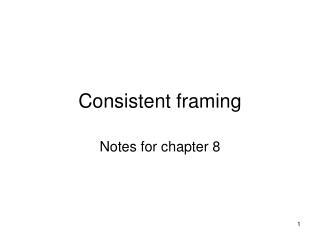DownloadDownload PresentationConsistent framing

# Consistent framing

Télécharger la présentation## Consistent framing

- - - - - - - - - - - - - - - - - - - - - - - - - - - E N D - - - - - - - - - - - - - - - - - - - - - - - - - - -
##### Presentation Transcript

1. Consistent framing Notes for chapter 8

2. Accounting and decisions • In accounting decision problems are often modified Fixed costs are not included • Often only incremental costs are considered • Costs are approximated • Profit maximization is often assumed • Cost minimization • Uncertainty is often not considered • Risk and risk premiums • Variance and covariance among projects • Time preferences are also disregarded • No discounting

3. More accounting • Product classification • Primary product • Secondary products • Scrap • Cost allocations • Transfer pricing • ABC costing

4. Rational Behavior • Intelligent, wise, and enlightened. • Economic setting pursue • Self-interest • Wealth • How do we describe rationality • How do we model rationality

5. Rational Behavior • Consistency • Complete and transitive ranking • Twostatements equivalent. • Rankingcompleteand transitive. • Existsa function on A, ω(a), • a`, a ́ ∈ A, ω(a`) ≥ ω(a ́) • Onlywhena` is ranked as good as a ́. • (The set A is finite) • Smoothness

6. A generic decision problem We want to maximize a generic function subject to some constraints of feasibility. This could take the form as:

7. Decision with two variables

8. Irrelevance of Increasing Transformations

9. Irrelevance of Increasing Transformations

10. Irrelevance of Increasing Transformations

11. Irrelevance of Increasing Transformations • Definition 19 Function T is an increasing transformation of function ω(a) if ω(a) > ω(â) if and only if T[ω(a)] > T[ω(â)] for every a and â in the domain of the original function • The solution to a decision problem is unaffected by an increasing transformation of the objective function.

13. Local search - Shadow prices Optimal choice is x = 4, y = 4

14. Component searches are possibleInteractions

15. Component searches are possible This reduces to: Then we get:

16. Component searches are possibleInteractions

17. Component searches are possible

18. Component searches are possible

19. Component search • When faced with an optimization problem of several variables we do component search when we solve the problem sequentially, by first optimizing with respect to one variable then the next etc.

20. Consistent Framing • 3 principles: • Irrelevance of Increasing Transformation • Local searches are possible • Component searches are Possible

21. Application of framing principles and cost functions

22. New framing!

23. Yet another framing! Using Component search this reduces to: Then we get:

24. Cost function I

25. Cost function II

26. Cost function III

27. Changed parameters

28. Cost function –Changed parameters

29. Frame I – Short Term

30. Frame II – Short Term

31. Frame II – Short Term • Short run cost: C(q1,q2)=60q1 +100q2 • No labor cost • New use of the accounting library • What we mean by cost – depends!

32. Cost terminology • Cost and benefit • F(z) = B(z) – C(z) • Separation always possible • Separation hardly unique • Relevant cost • Is simply the portion of the cost function that varies with the options at hand • Depends upon framing

33. Our objective • Are we maximizing profit? • Are we maximizing wealth? • Are we maximizing utility? • Are these different? • What happened to uncertainty? • How do we cope with uncertainty? • Is risk aversion part of our story?

34. Back to accounting • Cost function • Which products are included? • How is scrap accounted for? • Cost allocation • Are externalities accounted for? • Transfer pricing • Linear pricing – first order condition maintained? • ABC costing • Approximation of cost function?

35. Conclusions • Ease of analysis vs complete specification • Framing is this decision • Notion of cost follows frame • Cost allocation might be part of framing • Where did the problem set-up come from • Out of the blue • A handy and clever representation of the problem at hand • Professional judgment